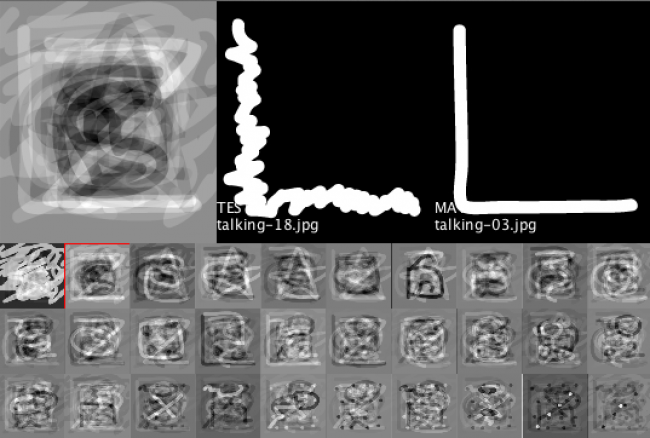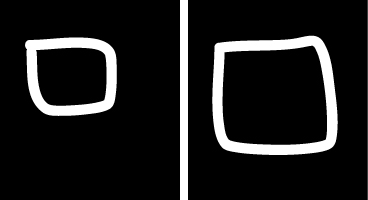#### Howdy, Stranger!

We are about to switch to a new forum software. Until then we have removed the registration on this forum.

# Pattern recognition

edited November 2014

I want to create a sketch that given a drawing (100% white over 100% black, thick stroke) would compare against a set of samples and provide the best match over a level of confidence. I've found good resources in this Daniels Shiffman's class notes: https://github.com/shiffman/Face-It

The application will have to run without connectivity, so I discarded Rekognition. I adapted an example using Eigenfaces-Processing library with my own set of drawing samples and test images with pretty good results:However I noticed that the library compares images 1 to 1 in size and rotation. I was wondering if there is a way of performing a pattern matching including scale and rotation, so these are recognized as similar images:Do you know of a library that does that? I would appreciate any help on this topic. Thanks in advance.

Tagged:

• I think you got very far indeed already...

scale

you scale the thing: when you measure the distance from the border to the 1st white pixel (measure from bottom and from top or from all 4 sides)

then on the left image distances are say 322 in sum, in the right 98 in sum.

then you need to scale the smaller by a certain value (maybe 322/98? Not sure)

for rotating

for rotating: when you have only 90° (or mirroring on itself) you could produce those ca. 3 images automatically and compare the result to the given image...

such things are done by neural networks, but this is hard too I guess, although there's a chapter in Shiffmanns book on that topic.

;-)

• edited November 2014

hello !

1) define the Xmin, Ymin, Xmax, Ymax of all your points

2) normalizes each values

``````point.x = (point.x - Xmin) / (Xmax - Xmin);
point.y = (point.y - Ymin) / (Ymax - Ymin);
``````

3) set a new position for your points, located from the center instead of using the topleft corner (then we will be able to rotate it), and rescale the values (multiply them by 20 for exemple, to get a 20x20 picture (then a more simple picture than original one)

``````float newScale = 20.0:
float halfScale = newScale / 2;

float dx = point.x - 0.5;
float dy = point.y - 0.5;
float d = sqrt(dx*dx+dy*dy) * halfScale ;
float angle = atan2(dy,dx);
point.x = halfScale  + cos(angle) * d;
point.y = halfScale  + sin(angle) * d;
``````

4)create an array of array of int, rotate your first 20x20 picture 360 times (for each degree) and register inside the arrays the différent point position. (actually, I think 360 time is too much, 50 times with rotation incremented by 360/50 should be enough)

5) take you second picture, for the comparison, grab its pixel-array

6) loop on you array of rotated-int-array and compute "the distance" (how much pixels doesn't match on the 2 pictures) , and register that distance in a object located in an ArrayList (one object by pixel, then 20x20 objects)

``````  int i,j,nbPixel = 20*20,nbRotation=50;
int[] rotatedShape;
int d;
for(i=0;i<nbRotation;i++){
rotatedShape = rotations[i];
d = 0:
for(j=0;j<nbPixel;j++){
if(rotatedShape[j] != secondShape[j]) d++
}
distanceList.get(i).distance = d;
}
``````

7) use Collections.sort to sort your arrayList by distance

`Collections.sort(distanceList);`

8) then, if the less distance found is less than a certain value, you can consider that the two pictures match.

``````if(distanceList.get(0).distance < distanceMin){
println("YEAH")
}
``````

Good luck !

• don't know about Shiffmanns book, he probably acts in a different way -